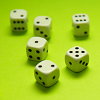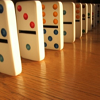#### You may also like### Consecutive Numbers

An investigation involving adding and subtracting sets of consecutive numbers. Lots to find out, lots to explore.### Roll These Dice

Roll two red dice and a green dice. Add the two numbers on the red dice and take away the number on the green. What are all the different possible answers?### Domino Square

Use the 'double-3 down' dominoes to make a square so that each side has eight dots.

# Coded Hundred Square

##### Age 7 to 11Challenge Level

Where could you start?
What might the first row of numbers look like? Why?
How might the highest number look different from the others?
What do you know about the multiples of 11?
What will be the same in each column?
What will be the same for the first nine numbers in each row?